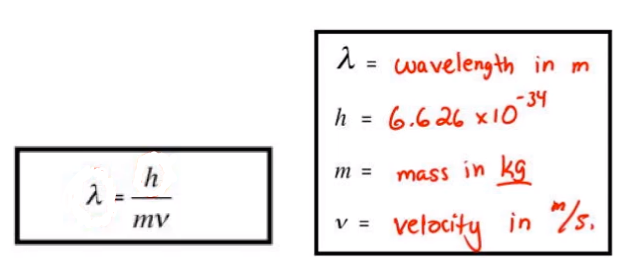# Problem: Calculate the velocities of electrons with de Broglie wavelengths of 1.0 x 10  2 nm and 1.0 nm.

###### FREE Expert Solution

Matter can behave as a wave and therefore will have a corresponding wavelength. The De Broglie Wavelength equation relates wavelength to velocity or speed.The constant stands for the Planck constant with a unit of m2 kg / s.

The mass of an electron is m= 9.109×1031 kg

81% (158 ratings)###### Problem Details

Calculate the velocities of electrons with de Broglie wavelengths of 1.0 x 10  2 nm and 1.0 nm.